Thread: Eric Dollard View Single Postt-rexSenior Member Join Date: Aug 2011 Location: In the bushes Posts: 422
Law of Electro-Magnetic Induction, Ten (1 of 2)

(1) Hysteresis is described as the separation in time between cause and effect. Cause and Effect relations can be expressed as points on a curve, normally this curve is a straight line. In the graphical representation of electronic devices this curve is called a Load Line. A straight line gives the condition of a direct relation between Cause and Effect.

As an example is the condition where the E.M.F., E, is the cause, and the Current, i, is the effect,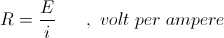The Proportionality, R, exists between Cause, E, and Effect, i, and here it is a Resistance. If the Cause, E, is given graphically as a vertical co-ordinate, the resulting Load Line is the graphical plot of the Proportionality between the Cause, E, and the Effect, i.

For the condition of a constant fixed resistance, R, in Ohm the Load Line is a straight line. The slope of this line is the instantaneous ratio of the E.M.F. to the Current, and it is constant anywhere along the line. No differential relation exists in that the ratio of any E.M.F. to its co-responding current is always the same value. It is a constant resistance, R.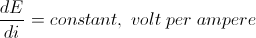This is known as a Linear relationship.

(2) Electrical devices such as Incandescent Lamps or Thyrites do not exhibit a direct relation between cause and effect. For example, the Lamp shows and Increasing Resistance for and Increasing Current, and a Thyrite shows a decreasing Resistance for an increasing applied E.M.F. Here the Load Line is no longer straight, but it is now curved in a parabolic form. The slope is no longer constant but varies with position along the curve. An E.M.F. and its co-responding Current have a different ratio than another E.M.F. and Current taken at another point on the curve. The variation of the slope represents the variation of Resistance,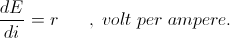Cause and Effect are now in Dis-Proportion to each other. Here Effect can become exaggerated by the cause and a sine wave of E.M.F. no longer gives rise to a sine wave of Current, distortion results. This is known as a Non-Linear relationship. Magnetic Saturation in Magnetic material is one such disproportional relationship, here between the M.M.F. and the Magnetic Induction, the Load Lines are known as the Saturation Curves of the Magnetic Material.

In both the Linear and the Non-Linear relation every E.M.F. has one and only one co-responding current. These exist at one unique point on the Proportionality Curve. The Relationship here is Uni-Valent, or single valued.

(3) Another more complex relation can exist between Cause, E, and Effect, i. In this relationship Cause and Effect become Dis-Joint. Here the curve for rising values is not the same curve as for falling values. In this situation the graphical expressions of the relation is no longer Linear, nor is it non-Linear, it is now an Elliptical relationship. In the Non-Linear relationship the limit in curvature is the straight line, here the curvature is Infinitesimal. Likewise for the Elliptical relationship, the limit in ellipticity is the full circle, a limiting case for Elliptical curvature. When the Load Line is a circle the Proportionality Factor of Resistance becomes the Dis-Proportionality Factor of Reactance.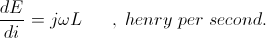Reactance is Resistance in constant variation at an angular rate of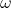. Here the Resistance is the Reaction of the Inductance to the constantly variable current,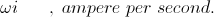(4) For the condition of the Elliptical Load Line, the point by point relationship is no longer uni-valent, for each Cause, or E.M.F., E, there exist two co-responding Effects, or Currents, i. These two Effects exist displaced in Time. Likewise, for each Effect, Current, i, there exists two co-responding Causes, E.M.F.s. These two Causes exist displaced in Time. Where it is the Linear or Non-Linear relationship is a Uni-Valent function, it is for the Circular and Elliptical relationships a Quadra-Valent function.

For the condition of the Linear and the Non-Linear relationships, As the Cause, or E.M.F. becomes smaller and smaller, likewise the Effect or Current becomes smaller and smaller. For both Proportionate and Dis-Proportionate relationships a zero Cause has a co-responding zero Effect. Both become zero together, Uni-Valent.

For the condition of the Circular and Elliptical relationships, these Quadra-Valent functions arrive at zero points in four locations on the curve, two for the Cause, E.M.F. and two for the Effect, Current. The two zero points for E.M.F. are displaced in Time as are the two zero points for Current, and all four zeros are displaced in Time from each other. Moreover here exists a unique situation where a Cause, E.M.F. can have zero Effect, Current, or and Effect, Current, can have no Cause, E.M.F. Cause and Effect are here Dis-Joint from each other. This condition can be called the Hysteretic Cycle of Proportionality.

(5) In its most general form, ruling out Non-Linearity, the Load Line can be considered a Circle rotating on an axis, this axis in the plane of the Circle and normal to its curvature, bisecting it. As this circle is turned on its axis it begins to contract into an ellipse. Continuing the rotation further, upon reaching one quadrant, 90 degrees, of rotation, the circle has completely contracted into a line with a slope of one, a 45 degree line. This quadrantal rotation represents the transformation from Reactance to Resistance. The angle by which the circle is displaced towards the line is called the Angle of Hysteresis,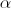.

In order to carry the angle,, beyond one quadrant one more transformation is required. Here the circle has a pair of rotational axes, these also in a quadrature relation, dividing the circle into four quadrants. As the angle,, passes beyond 90 degree the rotational axis is shifted to the quadrantal axis and it is inverted. As the line opens into an ellipse the position of this curve now travels in the opposite direction, the A.C. wave now rotates around the Load-Line in the opposite direction. Continuing to advance angle,, another 90 degrees, upon reaching two quadrants the line has opened up again into a full circle with a cyclic direction opposite to the circle at the start when the angle,, was zero. This now is a Negative Reactance. Where the first quadrant of rotation transformed Reactance into Resistance, the second of rotation transforms Resistance into Negative Reactance. Continuing to carry the rotational, or Hysteretic Angle,, beyond two quadrants, or 180° degrees, again contracts this counter-circle back into an ellipse. However the slope of this ellipse is now backwards, or negative, this as well as counter-cyclic. Upon reaching the next quadrant of rotation the ellipse has contracted into a line but now the line has a negative slope. Here is the unique situation where an increasing Cause, E.M.F. has a co-responding decreasing Effect, Current. Inversely, it is the greater the Effect, the less the Cause required to produce this Effect. This is a condition of Negative Resistance.
__________________
SUPPORT ERIC DOLLARD'S WORK AT EPD LABORATORIES, INC.

Purchase Eric Dollard's Books & Videos - 70% of the sale goes to Eric and EPD Laboratories: Eric Dollard Books & Videos
Donate by Paypal: Donate to EPD Laboratories